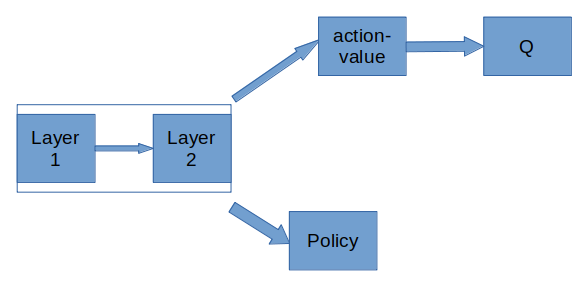# Stability of a 2-pass update through a shared layer?

Hey all! Say I’ve got a network like the followingTraining the RL algorithm, DDPG, comprises 2 steps:

OpenAI Algorithm Outline

I see a number of implementations online, but most do not have a shared layer so I don’t have a baseline to compare against.

Line 12 and 13

This portion describes how to compute the loss and gradient update through the first head (Q->action-value)

Line 14

This portion describes how to compute the loss and gradient update through the second head, Policy. I’ve coded it such that on every pass of the actor-critic I receive 2 outputs, the policy and the Q-value.

I’ve separated this into 2 optimization steps:

# Step 1

self.actor_critic.critic_optimizer.zero_grad()
_, y_hat_critic = self.actor_critic(s_batch, a_batch)
critic_loss = F.mse_loss(y_hat_critic, y_critic)
critic_loss.backward()
self.actor_critic.critic_optimizer.step()


# Step 2

self.actor_critic.actor_optimizer.zero_grad()
_, y_hat_policy = self.actor_critic(s_batch)
policy_loss = T.mean(-y_hat_policy)
policy_loss.backward()
self.actor_critic.actor_optimizer.step()


P.s I’ve verified that in the first case, the policy head’s gradient update is zero, and in the second, although a gradient is generated for the Q-value head, it is not applied.

However, I am facing an issue in terms of determining if the 2 pass update would cause the shared layer to run into stability issues. I’ve got self.custom_gradient_scaling_for_shared(self.actor_critic.parameters()) which determines if the update is for the shared layer, and if it is, it just scales the gradient.

Does anyone have insights into this?

I don’t think it necessarily is a problem, but if you disregard the update between step 1 and step 2 and the potentially different optimizer hyperparameters, you are effectively optimizing the shared weights to a sum of the two objective functions. Depending on the size relations of the two, this may make more or less sense.

Best regards

Thomas

P.S.: Is it intentional that you take the second output of actor_critic for both?

I don’t think it necessarily is a problem, but if you disregard the update between step 1 and step 2 and the potentially different optimizer hyperparameters, you are effectively optimizing the shared weights to a sum of the two objective functions

True, true! I was just wondering if this might pose a problem since, as we both mentioned, it’s optimizing over two objectives (hence my scaling the gradient update for the shared layers).

P.S.: Is it intentional that you take the second output of actor_critic for both?

Yeah, the first output is the output of the actor, and the second is the output of the critic. Our actor_critic has 2 “cases”:

1. self.actor_critic(state, action), the critic head uses the passed-in action to output the Q-value. (line 13, the LHS of the optimization eqn)

Q_{\phi}(s, a) where (s, a) are sampled from the replay buffer.

1. self.actor_critic(state) where we do not pass in an action. Here, we use our policy head to output a policy and we then output the Q-value from that (Note how in line 14, of the algorithm, we are taking the derivative of the Q-value but WRT the actor’s parameters)

Q_{\phi}(s, mu_{\theta}(a)). I needed to be careful with how I generated the gradient but I think I did it correctly.

The below is the code implementing “case 2”

Optimizer

params = list(self.shared_module.parameters()) + list(self.mu.parameters())


Forward pass in “case 2”

compressed: T.Tensor = self.shared_layer(state)
########################################
# Actor runs first
########################################
mu_val = self.mu(F.relu(compressed))
actions: T.Tensor = T.tanh(mu_val)
leq, geq = actions <= 1, actions >= -1
if not torch.all(leq * geq):
raise Exception("Our actions were out of the expected range")

########################################
# Critic runs now
########################################
action_value = F.relu(self.action_value(actions))
state_action_value = self.q(